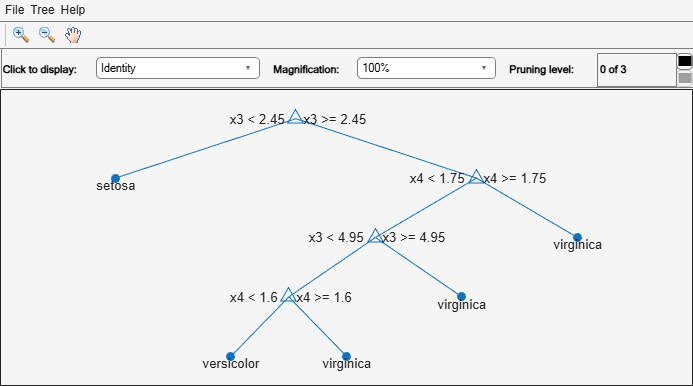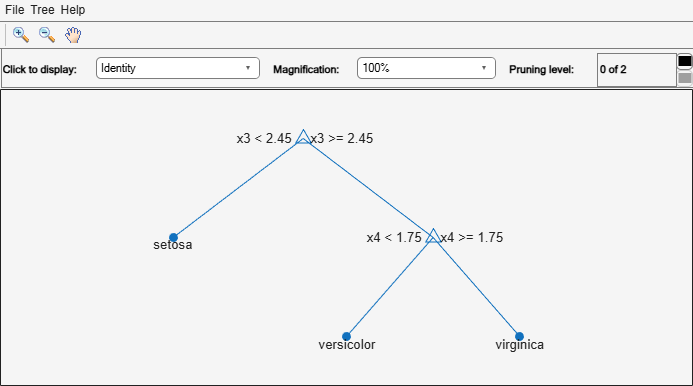Documentation

### This is machine translation

Mouseover text to see original. Click the button below to return to the English version of the page.

Note: This page has been translated by MathWorks. Click here to see
To view all translated materials including this page, select Country from the country navigator on the bottom of this page.

# loss

Classification error

## Syntax

``L = loss(tree,TBL,ResponseVarName)``
``L = loss(tree,TBL,Y)``
``L = loss(tree,X,Y)``
``L = loss(___,Name,Value)``
``````[L,se,NLeaf,bestlevel] = loss(___)``````

## Description

````L = loss(tree,TBL,ResponseVarName)` returns a scalar representing how well `tree` classifies the data in `TBL`, when `TBL.ResponseVarName` contains the true classifications.When computing the loss, `loss` normalizes the class probabilities in `Y` to the class probabilities used for training, stored in the `Prior` property of `tree`.```
````L = loss(tree,TBL,Y)` returns a scalar representing how well `tree` classifies the data in `TBL`, when `Y` contains the true classifications.```
````L = loss(tree,X,Y)` returns a scalar representing how well `tree` classifies the data in `X`, when `Y` contains the true classifications.```
````L = loss(___,Name,Value)` returns the loss with additional options specified by one or more `Name,Value` pair arguments, using any of the previous syntaxes. For example, you can specify the loss function or observation weights.```
``````[L,se,NLeaf,bestlevel] = loss(___)``` also returns the vector of standard errors of the classification errors (`se`), the vector of numbers of leaf nodes in the trees of the pruning sequence (`NLeaf`), and the best pruning level as defined in the `TreeSize` name-value pair (`bestlevel`).```

### Note

`loss` returns `se` and further outputs only when the `LossFun` name-value pair is the default `'classiferror'`.

## Input Arguments

expand all

Trained classification tree, specified as a `ClassificationTree` or `CompactClassificationTree` model object. That is, `tree` is a trained classification model returned by `fitctree` or `compact`.

Sample data, specified as a table. Each row of `TBL` corresponds to one observation, and each column corresponds to one predictor variable. Optionally, `TBL` can contain additional columns for the response variable and observation weights. `TBL` must contain all the predictors used to train `tree`. Multi-column variables and cell arrays other than cell arrays of character vectors are not allowed.

If `TBL` contains the response variable used to train `tree`, then you do not need to specify `ResponseVarName` or `Y`.

If you train `tree` using sample data contained in a `table`, then the input data for this method must also be in a table.

Data Types: `table`

Data to classify, specified as a numeric matrix. Each row of `X` represents one observation, and each column represents one predictor. `X` must have the same number of columns as the data used to train `tree`. `X` must have the same number of rows as the number of elements in `Y`.

Data Types: `single` | `double`

Response variable name, specified as the name of a variable in `TBL`. If `TBL` contains the response variable used to train `tree`, then you do not need to specify `ResponseVarName`.

If you specify `ResponseVarName`, then you must do so as a character vector or string scalar. For example, if the response variable is stored as `TBL.Response`, then specify it as `'Response'`. Otherwise, the software treats all columns of `TBL`, including `TBL.ResponseVarName`, as predictors.

The response variable must be a categorical, character, or string array, logical or numeric vector, or cell array of character vectors. If the response variable is a character array, then each element must correspond to one row of the array.

Data Types: `char` | `string`

Class labels, specified as a categorical, character, or string array, a logical or numeric vector, or a cell array of character vectors. `Y` must be of the same type as the classification used to train `tree`, and its number of elements must equal the number of rows of `X`.

Data Types: `categorical` | `char` | `string` | `logical` | `single` | `double` | `cell`

### Name-Value Pair Arguments

Specify optional comma-separated pairs of `Name,Value` arguments. `Name` is the argument name and `Value` is the corresponding value. `Name` must appear inside quotes. You can specify several name and value pair arguments in any order as `Name1,Value1,...,NameN,ValueN`.

Loss function, specified as the comma-separated pair consisting of `'LossFun'` and a built-in, loss-function name or function handle.

• The following table lists the available loss functions. Specify one using its corresponding character vector or string scalar.

ValueDescription
`'binodeviance'`Binomial deviance
`'classiferror'`Classification error
`'exponential'`Exponential
`'hinge'`Hinge
`'logit'`Logistic
`'mincost'`Minimal expected misclassification cost (for classification scores that are posterior probabilities)
`'quadratic'`Quadratic

`'mincost'` is appropriate for classification scores that are posterior probabilities. Classification trees return posterior probabilities as classification scores by default (see `predict`).

• Specify your own function using function handle notation.

Suppose that `n` be the number of observations in `X` and `K` be the number of distinct classes (`numel(tree.ClassNames)`). Your function must have this signature

``lossvalue = lossfun(C,S,W,Cost)``
where:

• The output argument `lossvalue` is a scalar.

• You choose the function name (`lossfun`).

• `C` is an `n`-by-`K` logical matrix with rows indicating which class the corresponding observation belongs. The column order corresponds to the class order in `tree.ClassNames`.

Construct `C` by setting `C(p,q) = 1` if observation `p` is in class `q`, for each row. Set all other elements of row `p` to `0`.

• `S` is an `n`-by-`K` numeric matrix of classification scores. The column order corresponds to the class order in `tree.ClassNames`. `S` is a matrix of classification scores, similar to the output of `predict`.

• `W` is an `n`-by-1 numeric vector of observation weights. If you pass `W`, the software normalizes them to sum to `1`.

• `Cost` is a K-by-`K` numeric matrix of misclassification costs. For example, ```Cost = ones(K) - eye(K)``` specifies a cost of `0` for correct classification, and `1` for misclassification.

Specify your function using `'LossFun',@lossfun`.

For more details on loss functions, see Classification Loss.

Data Types: `char` | `string` | `function_handle`

Observation weights, specified as the comma-separated pair consisting of `'Weights'` and a numeric vector of positive values or the name of a variable in `TBL`.

If you specify `Weights` as a numeric vector, then the size of `Weights` must be equal to the number of rows in `X` or `TBL`.

If you specify `Weights` as the name of a variable in `TBL`, you must do so as a character vector or string scalar. For example, if the weights are stored as `TBL.W`, then specify it as `'W'`. Otherwise, the software treats all columns of `TBL`, including `TBL.W`, as predictors.

`loss` normalizes the weights so that observation weights in each class sum to the prior probability of that class. When you supply `Weights`, `loss` computes weighted classification loss.

Data Types: `single` | `double` | `char` | `string`

`Name,Value` arguments associated with pruning subtrees:

Pruning level, specified as the comma-separated pair consisting of `'Subtrees'` and a vector of nonnegative integers in ascending order or `'all'`.

If you specify a vector, then all elements must be at least `0` and at most `max(tree.PruneList)`. `0` indicates the full, unpruned tree and `max(tree.PruneList)` indicates the completely pruned tree (i.e., just the root node).

If you specify `'all'`, then `loss` operates on all subtrees (i.e., the entire pruning sequence). This specification is equivalent to using `0:max(tree.PruneList)`.

`loss` prunes `tree` to each level indicated in `Subtrees`, and then estimates the corresponding output arguments. The size of `Subtrees` determines the size of some output arguments.

To invoke `Subtrees`, the properties `PruneList` and `PruneAlpha` of `tree` must be nonempty. In other words, grow `tree` by setting `'Prune','on'`, or by pruning `tree` using `prune`.

Example: `'Subtrees','all'`

Data Types: `single` | `double` | `char` | `string`

Tree size, specified as the comma-separated pair consisting of `'TreeSize'` and one of the following values:

• `'se'``loss` returns the highest pruning level with loss within one standard deviation of the minimum (`L`+`se`, where `L` and `se` relate to the smallest value in `Subtrees`).

• `'min'``loss` returns the element of `Subtrees` with smallest loss, usually the smallest element of `Subtrees`.

## Output Arguments

expand all

Classification loss, returned as a vector the length of `Subtrees`. The meaning of the error depends on the values in `Weights` and `LossFun`.

Standard error of loss, returned as a vector the length of `Subtrees`.

Number of leaves (terminal nodes) in the pruned subtrees, returned as a vector the length of `Subtrees`.

Best pruning level as defined in the `TreeSize` name-value pair, returned as a scalar whose value depends on `TreeSize`:

• `TreeSize` = `'se'``loss` returns the highest pruning level with loss within one standard deviation of the minimum (`L`+`se`, where `L` and `se` relate to the smallest value in `Subtrees`).

• `TreeSize` = `'min'``loss` returns the element of `Subtrees` with smallest loss, usually the smallest element of `Subtrees`.

By default, `bestlevel` is the pruning level that gives loss within one standard deviation of minimal loss.

## Examples

expand all

Compute the resubstituted classification error for the `ionosphere` data set.

```load ionosphere tree = fitctree(X,Y); L = loss(tree,X,Y)```
```L = 0.0114 ```

Unpruned decision trees tend to overfit. One way to balance model complexity and out-of-sample performance is to prune a tree (or restrict its growth) so that in-sample and out-of-sample performance are satisfactory.

Load Fisher's iris data set. Partition the data into training (50%) and validation (50%) sets.

```load fisheriris n = size(meas,1); rng(1) % For reproducibility idxTrn = false(n,1); idxTrn(randsample(n,round(0.5*n))) = true; % Training set logical indices idxVal = idxTrn == false; % Validation set logical indices```

Grow a classification tree using the training set.

`Mdl = fitctree(meas(idxTrn,:),species(idxTrn));`

View the classification tree.

`view(Mdl,'Mode','graph');`The classification tree has four pruning levels. Level 0 is the full, unpruned tree (as displayed). Level 3 is just the root node (i.e., no splits).

Examine the training sample classification error for each subtree (or pruning level) excluding the highest level.

```m = max(Mdl.PruneList) - 1; trnLoss = resubLoss(Mdl,'SubTrees',0:m)```
```trnLoss = 3×1 0.0267 0.0533 0.3067 ```
• The full, unpruned tree misclassifies about 2.7% of the training observations.

• The tree pruned to level 1 misclassifies about 5.3% of the training observations.

• The tree pruned to level 2 (i.e., a stump) misclassifies about 30.6% of the training observations.

Examine the validation sample classification error at each level excluding the highest level.

`valLoss = loss(Mdl,meas(idxVal,:),species(idxVal),'SubTrees',0:m)`
```valLoss = 3×1 0.0369 0.0237 0.3067 ```
• The full, unpruned tree misclassifies about 3.7% of the validation observations.

• The tree pruned to level 1 misclassifies about 2.4% of the validation observations.

• The tree pruned to level 2 (i.e., a stump) misclassifies about 30.7% of the validation observations.

To balance model complexity and out-of-sample performance, consider pruning `Mdl` to level 1.

```pruneMdl = prune(Mdl,'Level',1); view(pruneMdl,'Mode','graph')```expand all

Download ebook